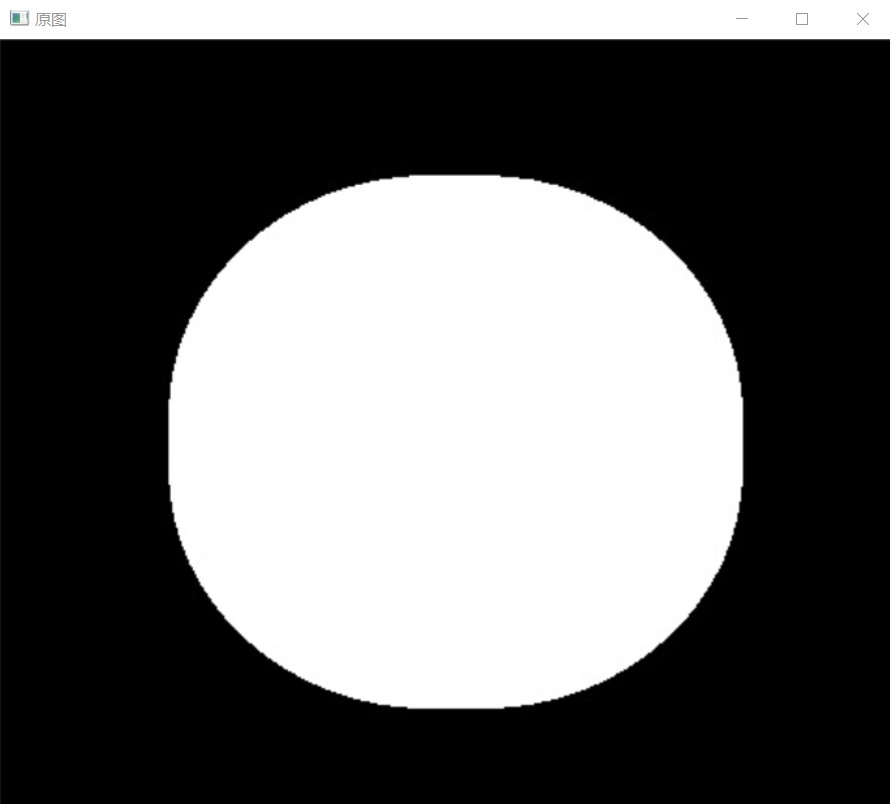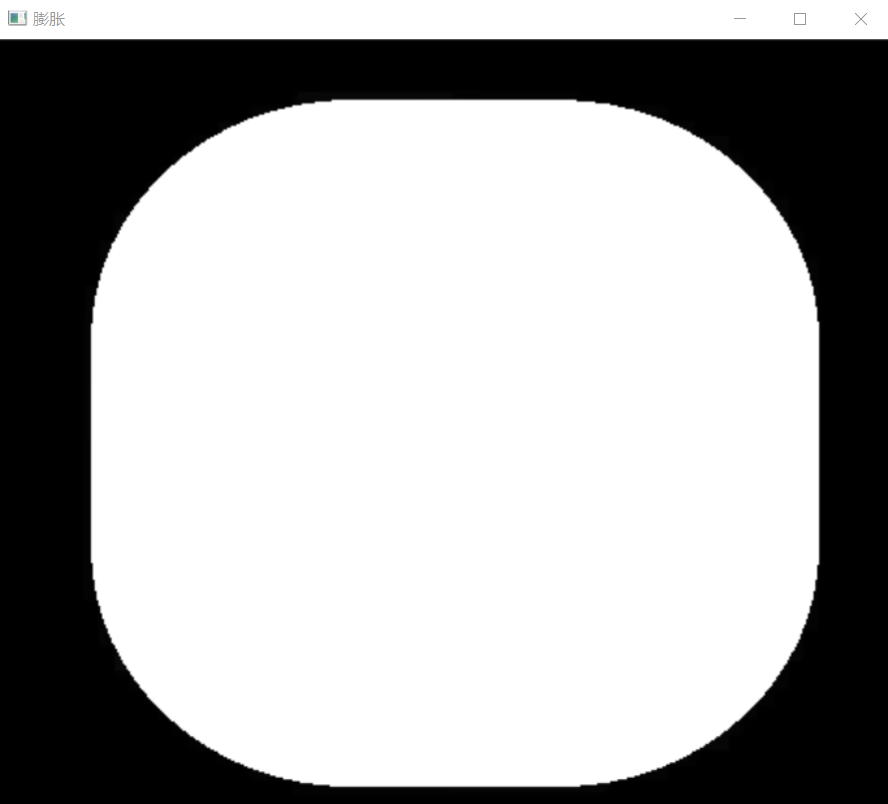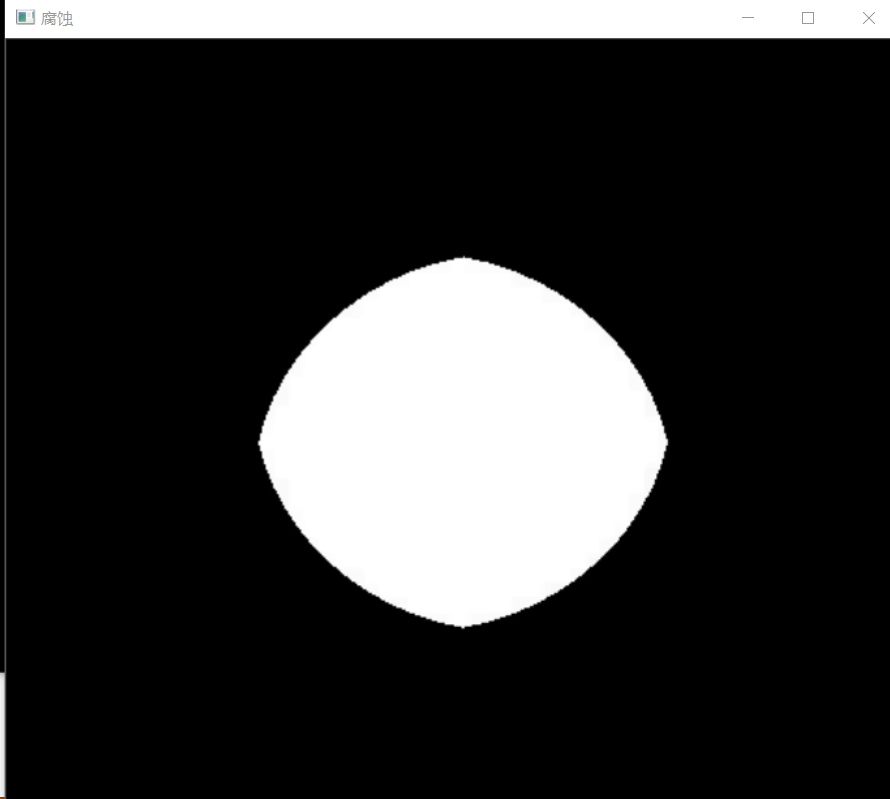# opencv——形态学深究（分析和应用）

## 摘要：

• 膨胀和腐蚀（膨胀区域填充，腐蚀分割区域）
• 开运算和闭运算（开运算去除噪点，闭运算填充内部孔洞）
• 击中与击不中
• 顶帽变换，黑帽变换

🧡在讲各种形态学操作之前，先来看看结构元素：

OpenCV里面的API介绍：

```Mat kernel = getStructuringElement(int shape,Size ksize，Point anchor）;
shape   //结构元素的定义：形状 (MORPH_RECT \MORPH_CROSS（交叉形） \MORPH_ELLIPSE)；
ksize   //结构元素大小；
anchor  //锚点 默认是Point(-1, -1)意思就是中心像素,也可以自己指定```

• 膨胀就是使图像中高亮部分扩张，效果图拥有比原图更大的高亮区域（是求局部最大值的操作）
• 腐蚀是原图中的高亮区域被蚕食，效果图拥有比原图更小的高亮区域（是求局部最小值的操作）

1、消除噪声
2、腐蚀分割(isolate)出独立的图像元素，膨胀在图像中连接(join)相邻的元素。
3、寻找图像中的明显的极大值区域或极小值区域
4、求出图像的梯度

opencv中膨胀/腐蚀API:(两者相同）

```void dilate/erode( const Mat& src,   //输入图像（任意通道的）
Mat& dst,   //输出图像
const Mat& element,   //结构元素
Point anchor=Point(-1,-1),   //中心位置锚点
int iterations=1,   //操作次数。省略时为默认值1。
int borderType=BORDER_CONSTANT,   //边缘填充类型
const Scalar& borderValue    //填充值（默认即可）
)```

opencv实现：

```    Mat src1 = imread("D:/opencv练习图片/腐蚀膨胀.png");
Mat src_erode, src_dilate;
imshow("原图", src1);
Mat kernel = getStructuringElement(MORPH_RECT, Size(60, 60), Point(-1, -1));
erode(src1, src_erode, kernel, Point(-1, -1),2);
dilate(src1, src_dilate, kernel, Point(-1, -1),2);
imshow("腐蚀", src_erode);
imshow("膨胀", src_dilate);```1️⃣腐蚀操作的原理就是求局部最小值的操作，并把这个最小值赋值给参考点指定的像素。这样就会使图像中的高亮区域逐渐减少。

2️⃣膨胀操作的原理就是求局部最大值的操作，并把这个最大值赋值给参考点指定的像素。这样就会使图像中的高亮区域逐渐增长。

```void morphologyEx(
InputArray src,            //输入图像
OutputArray dst,            //输出图像
int op,            //形态学运算类型
InputArray kernel,           //结构元素
Point anchor = Point(-1,-1), //锚点
int iterations = 1,          //运算次数
int borderType = BORDER_CONSTANT,
)```

• MORPH_ERODE（腐蚀）
• MORPH_DILATE（膨胀）
• MORPH_OPEN（开运算）
• MORPH_CLOSE（闭运算）
• MORPH_TOPHAT（顶帽运算）
• MORPH_BLACKHAT（底帽运算）
• MORPH_HITMISS（击中与击不中）

🧡开运算和顶帽运算

opencv实现：

```    Mat src1 = imread("D:/opencv练习图片/开运算.png");
Mat src_open, src_tophat;
imshow("原图", src1);
Mat kernel = getStructuringElement(MORPH_RECT, Size(7, 7), Point(-1, -1));
morphologyEx(src1, src_open, MORPH_OPEN, kernel,Point(-1, -1));//开运算
morphologyEx(src1, src_tophat, MORPH_TOPHAT, kernel, Point(-1, -1));//顶帽运算
imshow("开运算", src_open);
imshow("顶帽运算", src_tophat);```

闭运算和底帽运算

opencv实现：

```    Mat src1 = imread("D:/opencv练习图片/闭运算.png");
Mat src_close, src_blackhat;
imshow("原图", src1);
Mat kernel = getStructuringElement(MORPH_RECT, Size(7, 7), Point(-1, -1));
morphologyEx(src1, src_close, MORPH_CLOSE, kernel,Point(-1, -1));//闭运算
morphologyEx(src1, src_blackhat, MORPH_BLACKHAT, kernel, Point(-1, -1));//底帽运算
imshow("闭运算", src_close);
imshow("底帽运算", src_blackhat);```

形态学梯度（求二值图边缘)

• 基本梯度（图像膨胀与腐蚀操作之间的差值）
• 内梯度（输入图像与腐蚀之间的差值）
• 外梯度（膨胀与输入图像之间的差值）

opencv实现：

```    Mat src1 = imread("D:/opencv练习图片/腐蚀膨胀.png");
imshow("原图", src1);
Mat kernel = getStructuringElement(MORPH_RECT, Size(7, 7), Point(-1, -1));
//基本梯度
//外梯度
morphologyEx(src1, src_dilate, MORPH_DILATE, kernel, Point(-1, -1));
subtract(src_dilate, src1, src_exter);//求差值
imshow("外梯度", src_exter);
//内梯度
morphologyEx(src1, src_erode, MORPH_ERODE, kernel, Point(-1, -1));
subtract(src1, src_erode, src_inter);
imshow("内梯度", src_inter);```

Hit-miss算法步骤(两次腐蚀，求交集）：

• 首先，建立一个比B大的结构元素B1；使用B1对图像A进行腐蚀，得到图像假设为A_B1;
• 其次，用B减去B1，从而得到结构元素B2(B2-B)；使用B2对图像A的补集进行腐蚀，得到图像假设为A_B2;
• 然后，A_B1与A_B2取交集；得到的结果就是B的位置。

opencv实战（利用击中与击不中，提取网绳的结点位置）：

```Mat src = imread("D:/opencv练习图片/击中与击不中.png");
imshow("原图", src);
// 二值图像
Mat gray, binary, hitImg;
cvtColor(src, gray, COLOR_BGR2GRAY);
//高斯滤波
Mat gauss;
GaussianBlur(gray, gauss, Size(5, 5), 0, 0);
//二值化
threshold(gauss, binary, 0, 255, THRESH_BINARY_INV | THRESH_OTSU);
imshow("binary", binary);
// 定义结构元素
Mat se = getStructuringElement(MORPH_CROSS, Size(11, 11), Point(-1, -1));
// 击中击不中
morphologyEx(binary, hitImg, MORPH_HITMISS, se);
imshow("击中击不中", hitImg);
//膨胀一下
Mat openImg;
Mat kern2 = getStructuringElement(MORPH_RECT, Size(3, 3));
morphologyEx(hitImg, openImg, MORPH_OPEN, kern2, Point(-1, -1), 2);
imshow("dilate", openImg);
//寻找轮廓
vector<vector<Point>>contours;
vector<Vec4i>hie;
findContours(openImg, contours, hie, RETR_TREE, CHAIN_APPROX_SIMPLE, Point());
for (size_t i = 0; i < contours.size(); i++)
{
double area = contourArea(contours[i]);
if (area < 20.0)continue;
Rect rect = boundingRect(contours[i]);
rectangle(src, rect, Scalar(0, 0, 255), 1, 8);

}
// 显示
imshow("结果", src);```